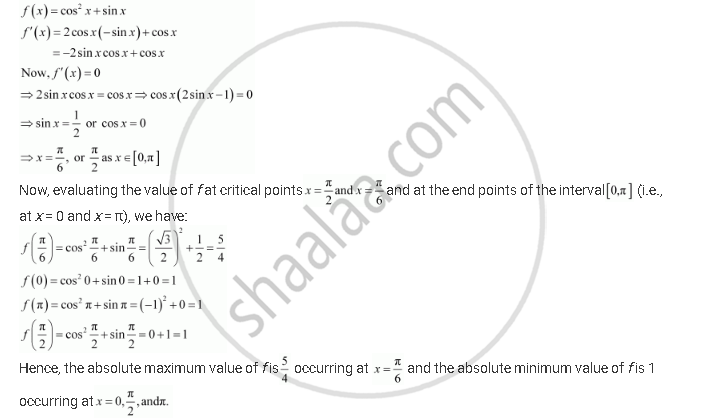Share

# Find the Absolute Maximum and Minimum Values of the Function F Given By F (X) = Cos2 X + Sin X, X ∈ [0, π] - CBSE (Commerce) Class 12 - Mathematics

#### Question

Find the absolute maximum and minimum values of the function f given by f (x) = cos2 x + sin x, x ∈ [0, π]

#### SolutionIs there an error in this question or solution?

#### APPEARS IN

NCERT Solution for Mathematics Textbook for Class 12 (2018 to Current)
Chapter 6: Application of Derivatives
Q: 14 | Page no. 243

#### Video TutorialsVIEW ALL 

Solution Find the Absolute Maximum and Minimum Values of the Function F Given By F (X) = Cos2 X + Sin X, X ∈ [0, π] Concept: Maxima and Minima.
S